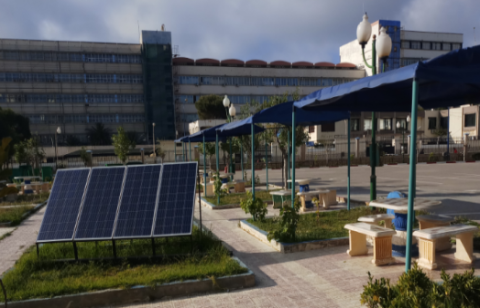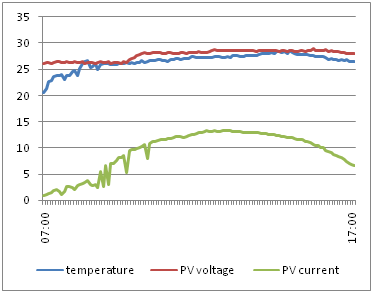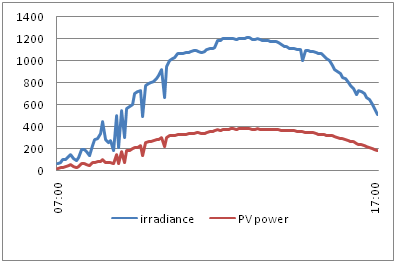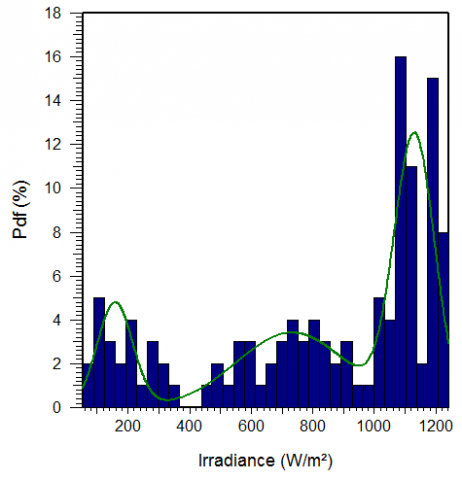# Estimation of Photovoltaic Energy Conversion Using Mixed Weibull Distribution

Estimation of Photovoltaic Energy Conversion Using Mixed Weibull Distribution
Institute of Electrical and Electronic Engineering, University of Boumerdes, Boumerdes 35000, Algeria

Department of Power and Control, University of Boumerdes, Boumerdes 35000, Algeria

Corresponding Author Email:
r.medjoudj@univ-boumerdes.dz
Page:
385-391
|
DOI:
https://doi.org/10.18280/jesa.530309
27 April 2020
|
Accepted:
20 May 2020
|
Published:
31 July 2020
| Citation

OPEN ACCESS

Abstract:

The uncertainty in the potential of electricity generation, due to the natural variation of solar radiation as daily changes, is one of the important challenges for the photovoltaic technology. This paper presents a stochastic modeling and analysis of the measured electrical parameters of a photovoltaic system, irradiance and the power generation under a spring day at Boumerdes location (Algeria). A comparison study between the sunny day and cloudy day is introduced to show the effect of the variation of irradiance and the temperature on the energy conversion. Stochastic modeling and analysis are made using mixed Weibull distribution, its parameters are estimated based on the maximum likelihood method. The obtained results in this work are; the estimated mean values of the irradiance, the power generation of the PV system, and the cumulative probability function of the energy conversion. The mean objective is to predict a generated power, for the adequate design and sizing of the PV array.

Keywords:

photovoltaic system, stochastic modeling, data analysis, power generation, mixed Weibull distribution

1. Introduction

A photovoltaic power system can be used to provide alternative and inexhaustible source of electrical power to our homes through the direct conversion of solar irradiance into electricity. The process of acquiring a photovoltaic power system involves designing, selecting and determining the specifications of the different components employed in the system. The success of this process depends on a variety of factors such as geographical location, weather condition, solar irradiance, and load profile. Photovoltaic system still has important challenges; one of them is the perception as an energy source with a prolonged investment return and unreliable. This is caused by the uncertainty in the potential of electricity generation, due to the natural variation of solar radiation between one area to another, as well as daily changes in climatic factors for a specific location where the PV panel is installed . Several Researches have proposed methods to estimate the potential energy based in the solar radiation data as the Hotel’s model [2, 3]. Some theoretical models for estimating the potential of solar electricity have been proposed [4, 5], and their analysis based on stochastic models of Weibull and Rayleigh using Probability Density Function approach to evaluate and discuss the Energy wind potential are developed . Whereas wind speed data has been statistically analyzed using Weibull distribution to find out wind energy conversion characteristics of Hatiya Island in Bangladesh . Two important parameters like Weibull shape factor and Weibull scale factor have been calculated by four different methods and the obtained results are presented .The principal laws used in mathematical modeling and analysis of data are: the exponential law, Weibull law, Gumbell law, the extreme values law, normal law and log-normal law, Weibull and log-normal distributions are the most used in engineering applications [9, 10]. One can usefully refer to the works of Nelson  and Lawless  that provide practical information on data processing. The effects related to long term weathering and degradation of the module package resulting in a degradation of the module performance are modeled using exponential and Weibull distribution . The 24-hour ahead forecasting performance of two methods based on Artificial Neural Network commonly used for the prediction of the power output of photovoltaic systems are compared .

Using the example of the town of Kamyshin in Volgograd oblast, the sufficiency of required actinometric information is analyzed for further solar-engineering calculations and the data accuracy and limits of their applicability are estimated . However, a hybrid method (PHANN – Physic Hybrid Artificial Neural Network is adopted to enhance the energy forecast combining both the deterministic Clear Sky Solar Radiation Algorithm (CSRM)and the stochastic ANN method is introduced .

Potential estimation and planning of electric power generation from solar energy available is the determining factor for investing in photovoltaic generation. Therefore, it is necessary to have the measurement instruments for solar radiation, temperature and electrical parameters of the PV system representing the current and the voltage [17, 18]. Adequate design and sizing of the PV array require also, the prediction of the power conversion to estimate the number of the components constituting the system (panels). For that Analysis of the parameters measured from the PV array installed in institute of electrical engineering and electronics Boumerdes University is introduced, based on stochastic modeling using mixed Weibull distribution. The mean Values of irradiance and power conversion are estimated to help investors to take good decisions on the sizing and design of PV systems in such location. The outline of the paper is as follows. In Section 2, a Weibull and mixed Weibull distributions for modeling and analyzing data of irradiance and the power generation are described. The experimental case study is presented in the section 3, where the electrical parameters, irradiance and temperature are measured and analyzed. A stochastic analysis of the real data concerning the potential irradiance and the power generation is introduced in the section 4, and the obtained results are also discussed. Section 5 summarizes the conclusions of this paper.

2. Statistical Models

The principal laws used in statistical modeling and data analysis are: the exponential law, Weibull law, Gumbell law, the extreme values law, normal law and log-normal law. Mixed Weibull Distribution is used when the data represent a non-homogeneous population.

2.1 Weibull statistical model

Weibull distribution is one of the most widely used distributions to represent the models. It is a versatile distribution that can take on the characteristics of other types of distributions; based on the value of the shape parameter β. The probability density function (PDF) and cumulative density function (CDF) are mathematical functions that describe the distribution. The PDF and CDF of the Weibull distribution are represented mathematically or on aplot where the x-axis represents the variable, and the y-axis represents the function.

The 2-parameter Weibull PDF is given by:

$P\left(P_{w}\right)=1-\exp \left[-\left(\frac{P_{w}}{\eta}\right)^{\beta}\right]$           (1)

$P($Irr$)=1-\exp \left[-\left(\frac{\operatorname{Irr}}{\eta}\right)^{\beta}\right]$        (2)

A detailed description of this theory can be found in references [10, 11]. Eq. (2) may be transformed in the following form:

$\log \left(\ln \left(\frac{1}{1-P\left(P_{w}\right)}\right)\right)=\beta \log \left(P_{w}\right)-\beta \log (\eta)$

If the observed phenomenon follows the two parameters Weibull model, the graph of the irradiance and the power in logarithmic scale on x-axis and the value

$\ln \left(\frac{1}{1-P\left(P_{w}\right)}\right)$

in logarithmic scale on y-axis must be a straight-line with a slope ꞵ. We note that for

$\operatorname{Irr}=\eta$

$P(\operatorname{Irr})=1-e^{-1}=63.2 \%$

$P\left(P_{w}\right)=1-e^{-1}=63.2 \%$

This is equivalent to the mean of a normal distribution. This typical value Irr and Pw of mean irradiance and mean power generation will be extracted from the following data and discussed later.

2.1.1 Mixed Weibull distribution

A mixed distribution comprises two or more distributions. Mixture arises when the population of interest contains two or more non homogeneous subpopulations. Such cases occur frequently in practice. The substandard subpopulation fails in early time, but the good one survives considerably longer. In addition to the mixture of good and bad products, a manufacturing process fed with components from different suppliers usually produces non homogeneous subpopulations.

A mixture of two distributions is usually of most interest. It is perhaps the most common mixed distribution in practice because of its inherent flexibility . The PDF of the mixed Weibull distributions f1(t) and f2(t) is:

$f(t)=\rho f_{1}(t)+(1-\rho) f_{2}(t)$        (3)

\begin{aligned} f(t)=& \rho \frac{\beta_{1}}{\eta_{1}^{\beta_{1}}} t^{\beta_{1}-1} \exp \left[-\left[\frac{t}{\eta_{1}}\right]^{\beta_{1}}\right] +(1-\rho) \frac{\beta_{2}}{\eta_{2}^{\beta_{2}}} t^{\beta_{2}-1} \exp \left[-\left[\frac{t}{\eta_{2}}\right]^{\beta_{2}}\right] \end{aligned}        (4)

The associated CDF is:

$F(t)=\rho F_{1}(t)+(1-\rho) F_{2}(t)$         (5)

$F(t)=1-\exp \left[-\left[\frac{t}{\eta_{1}}\right]^{\beta_{1}}\right]+(1-\rho) \exp \left[-\left[\frac{t}{\eta_{2}}\right]^{\beta_{2}}\right]$     (6)

2.2 Maximum likelihood method fitting

The likelihood method estimation is a well-known method for estimating the parameters of a statistical distribution. It has set up a numerical method for finding the two parameters of a Weibull distribution by using a likelihood function L :

$P(\eta, \beta)=\prod_{i=1}^{N} \frac{\beta}{\eta}\left(\frac{V_{i}}{\eta}\right)^{\beta-1} \exp \left[-\left(\frac{V_{i}}{\eta}\right)^{\beta}\right]$        (7)

By looking for maximum likelihood estimation on Eq. (7), we have to solve:

$\frac{\delta \log (L(\eta, \beta))}{\delta \eta}=0$ and $\frac{\delta \log (L(\eta, \beta))}{\delta \beta}=0$

3. Experimental Case Study

The circuit is designed to be used a small stand-alone system which can be extended. It consists of four PV modules, a MPPT charge controller, a single-phase inverter, two batteries and a load. The ENG36P100W Photovoltaic module is used in the implementation, which is made of 36 poly-crystalline cells connected in series. The Table 1 presents the characteristics of the solar module under standard test conditions (STC) from the data sheet. Since the maximum voltage from each module is 18.9 V, it needs to be increased to exceed 24 volts at the MPPT solar entrance. To do that, each two PV modules are connected in series, then the two strings in parallel.

Table 1. 100W PV module characteristics under STC

 Pmax Voc Isc Vmpp Impp NOCT TCVoc TCIsc TCPmax 100W 22.6V 5.53A 18.9V 5.3A -45% -0.32% 0.05% 0.45%

3.1 Data acquisition and analysis

The data acquisition system is used to measure the irradiance, temperature, current and voltage; then send them to be processed. The system consists of two circuits: The first one is fixed on the PV array to measure the irradiance and temperature using a photodiode and an IC temperature sensor (LM 35), and then send them to the main circuit. The latter is composed of Hall-effect and voltage measurement sensors which are responsible for measuring the current and the voltage respectively. The implemented circuits are represented in the following Figures 1-2:

## 1.pngFigure 1. PV array

## 2.pngFigure 2. Power circuit

Figure 3, Figure 4, Figure 5 and Figure 6 demonstrate how the weather affects the irradiance, which keeps changing in cloudy days due to the change in the density of the clouds. In sunny days, the irradiance exceeds 1200W/m².

However, in cloudy days it keeps varying between 300 to 600W/m².

The Figure 3 illustrates the measured temperature, current and voltage generated by the PV system during the sunny day. The temperature is approximately stable with small variation in the first few hours of the day. However, the MPPT T40 charge controller uses the constant voltage method which leads to fixed voltage values. The Figure 4 shows the irradiance and the power generation changes during that day, the both curves take almost the same profile.

From the Figure 6, the profile of the power generation calculated from the measured current and the voltage generated by the PV system, follows the profile of the irradiance. Since power is the product of the current and the voltage (the voltage is approximately stable), the change in power is propotional to the change in current where the main factor controlling the power and the current change is the irradiance.The power generated by the PV array varies from 25 W to reach a peak of 384W in a sunny day, but in cloudy days the power keeps varying from 20W to 200W only.

## 3.pngFigure 3. Measured parameters for sunny day

## 4.pngFigure 4. Irradiance and PV Power for sunny day

## 5.pngFigure 5. PV measured parameters for cloudy day

## 6.pngFigure 6. Irradiance and PV Power for cloudy day

4. Stochastic Analysis of Irradiance and Power Generation Data

The stochastic analysis of the real data concerning the potential irradiance of two different days on term of climatic changes, allows for the rechearchers and investors to understand the behaviour of such natural event, to predict the uncertenty power generation.

4.1 Irradiance and power generation analysis for the sunny and cloudy days

For the irradiance data , the kolmogorov-Smirnov Test (KS) comfirms the weibull distribution with 8 parameters (three subpopulation data), because the p-Value greater than or equal to 0.05. The coefficient of determination R²=0.991 for the fitted distribution is good. The same test is done for the power generation data and KS test confirms the Weibull distribution with 8 parameters. The coefficient of determination R²=0.987 is good.  The parameters are given in the Table 2.

A stochastic analysis of irradiance and PV power generation was made using the Weibull model with eight parameters. For estimating the scale and the shape parameters the likelihood technique is used. The Figures 7 and 8 below show the probability Pi versus experimental measured values of irradiance and PV power generation. They represent the results obtained for the comparison study of the sunny and cloudy days. The experimental data, the relevant distribution of power, its parameters and its cumulative density function that correspond to the data are essential elements, to determine the generation powers probability during that day.

Table 2. Mixed Weibull Parameters for sunny and cloudy days

 Parameters Irradiance W/m² Power generation (W) Cloudy Day Sunny Day Cloudy Day Sunny Day β1 1.91 2.43 2.9 2.52 η1 385 226.1 35.4 81 ρ1 0.40 0.22 0.37 0.26 β2 2.83 5.56 9.2 6.56 η2 540 840 84.4 290.1 ρ2 0.43 0.29 0.40 0.28 β3 34.2 15.9 33.9 19.1 η3 570 1156 181 362.3 1-(ρ1+ ρ2) 0.17 0.49 0.17 0.46

## 7.pngFigure 7. Weibull diagram for sunny and cloudy days

## 8.pngFigure 8. Weibull diagram for sunny and cloudy days

The mean irradiance and Power generation are deduced from the values of the probabilities when they are equal to 63.2%. The mean value of the irradiance and power generation are 485.4W/m², 143.95W respectively during the cloudy day. During the sunny day the mean values of the irradiance and power are respectively: 923W/m² and 307.9.

The Figures 9 and 10 show the Weibull density of probability for all the irradiance ranges observed during the sunny and cloudy days. It is observed that the irradiances are in three different ranges. Weibull distribution with eight parameters seems to give a reasonable fit to observed irradiances of sunny and cloudy days.

## 9.pngFigure 9. Density function for sunny day

## 10.pngFigure 10. Density function for Sunny day

## 11.pngFigure 11. Power generation probabilities during the sunny day

## 12.pngFigure 12. Power generation probabilities during the sunny day

The probabilities of having the energy conversion intervals are given in the Figures 11, 12. The objective is to show the important variation on the energy conversion during the day due to the weather changing. For the cloudy day, the probability to generate the power between 150 to 200W is equal to 0.42, and it is considered the high probability, while during the sunny day, the probability of producing the power between 300W to 400W is equal to 0.337, and the PV array is supposed to produce 400W under STC.

4.2 Irradiance and power generation analysis of eight successive days of month May 2019

During this period, the data collected in the real case study represents the different electrical parameters of current, voltage and irradiance and temperature. The power generation is calculated from the curent and the voltage generated by the PV system.

The Figure 13 illustrates the solar radiation and the energy conversion of PV system installed at Boumerdes location, during 8 successive days. Almost 4 days are cloudy and 4 days are sunny, and the mean value of the power generation is equal to 235W, while the installed system is supposed to generate 400W under STC.

By testing the Weibull distribution with eight parameters (the nonh-omoginous population of data is devided to three homoginous subpopulations), this latter is validated by the Kein test. The Weibull parameters are estimated based on the least square method, these parameters are represented in the following Table 3.

Table 3. Mixed Weibull parameters of the data

 Parameters Irradiance W/m² Power generation (W) β1 2.43 2.52 η1 226.1 81 ρ1 0.22 0.26 β2 5.56 6.56 η2 840 290.1 ρ2 0.29 0.28 β3 15.9 19.1 η3 1156 362.3 1-(ρ1+ ρ2) 0.49 0.46

## 13.pngFigure 13. Irradiance and power generation for 8 days

## 14.pngFigure 14. Weibull diagram for 8 days

The Figure 14 represents the Weibull probability plots of the irradiance and the power generation for all the period of the study. The mean irradiance in Boumerdes city location (Algeria) is equal to 741.6W/m² and the mean power generation is equal to 235.47W, for the PV array of 400W under standard test condition.

4.3 Discussion

In this work the measured data of irradiance and electrical parameters are analysed and modeled by using stochastic models. The profile of the energy conversion follows exactly the profile of the irradiance, where it is considered random variable, for why it is important to analyse that natural phenomena with stochastic models. As a result, the real conversion efficiency is lower almost 40% than conversion efficiency under standard test conditions (T=25°C, E=1000W/m²). The energy generated by the PV array of 400W for all the period of the study is equal to 235W, and a maximum power generated at Boumerdes location, under a good condition in the month of May, which are: 1267W/m² irradiance and 27℃ is 384 W.

This difference between the real power generation and the supposed Power generation under STC is due to the climatic changes. For example, if the investor or practiciener needs the power supply with Photovoltaic technology of 400W as a power demand, at this location, the number of the panels needed for that system is seven. The PV power system to install is almost 700W.

5. Conclusion

One of the determining factors for investing in photovoltaic generation is having a potential estimation and planning of electric power generation from solar energy available. Therefore it is necessary to have a practical method to estimate this potential for a specific location. In this paper, a stochastic model has been validated to estimate the nature variation of the solar radiation and the power generation by the PV array. This model was based on the real data of the daily variation of the irradiance and temperature, and the measured electrical parameters. A variation on the potential of solar radiation during the period of the study has been demonstrated, particularly the real energy conversion efficiency has been lower almost 40% than STC efficiency in Boumerdes city location (Algeria). Photovoltaic System must be reliable enough to ensure that the production and process engineering processes they serve can function efficiently. This is only possible if the planning decisions made on the design and sizing meet all the process requirements for power generation in a technically optimum and efficient way. For that this work allows for engineers to estimate potential energy and providing certainty to PV systems investment.

Nomenclature
 P(PW) Probability of having PW Power generation P(Irr) Probability of having Irr Potential radiation Voc Open circuit voltage Isc Short circuit current STC Standard test condition Pmax Maximum Power at STC NOCT Nominal operating cell temperature MPP Maximum power point MPPT Maximum power point tracking PV Photovoltaic TCVoc Temperature coefficient of Voc TCPmax Temperature coefficient of Pmax TCIsc Temperature coefficient of Isc Greek symbols β Shape parameter of Weibull distribution η Scale parameter of Weibull distribution ρ Portion probability
References

 Herrera, C.M., Sánchez, F.H., Bañuelos, M.F. (2014). Practical method to estimate energy potential generated by photovoltaic cells: Practice case at Merida City. Energy Procedia, 57: 245-254. https://doi.org/10.1016/j.egypro.2014.10.029

 Hottel, H.C. (1976). Simple model for estimating the transmittance of direct solar radiation through clear atmospheres. Solar Energy, 18(2): 129-134. https://doi.org/10.1016/0038-092X(76)90045-1

 Yousuf, M.U., Siddiqui, M., ur Rehman, N. (2018). Solar energy potential estimation by calculating sun illumination hours and sky view factor on building rooftops using digital elevation model. Journal of Renewable and Sustainable Energy, 10(1): 010401. https://doi.org/10.1063/1.4997888

 Súri, M., Huld, T., Dunlup, E., Ossenbrink, H. (2007). Potential of electricity generation in the European Union member states and candidate countries. Solar Energy, 81(10): 1295-1305. https://doi.org/10.1016/j.solener.2006.12.007

 Pillai, I., Banerjee, R. (2009). Renewable energy in India: Status and potential. Energy, 34(8): 970–980. https://doi.org/10.1016/j.energy.2008.10.016

 Bidaoui, H., El Abbassi, I., El bouardi, A., Darcherif, A. (2019). Wind speed data analysis using Weibull and Rayleigh distribution functions, case study: Five cities Northern Morocco. The 12th international conference interdisciplinary in engineering. Procedia Manufacturing 32: 786-793. https://doi.org/10.1016/j.promfg.2019.02.286

 Ali, A., Lee, S.M., Jang, C.M. (2018). Statistical analysis of wind characteristics using Weibull and Rayleigh distributions in Deokjeok-do Island Incheon, South Korea. Renewable Energy, 123: 652-663. https://doi.org/10.1016/j.renene.2018.02.087

 Azad, A.K., Rasul, M.G., Alam, M.M., Ameer Uddin, S.M., Mondal, S.K. (2014). Analysis of wind energy conversion system using Weibull distribution. 10th International Conference on Mechanical Engineering, ICME 2013, Procedia Engineering, 90: 725-732. https://doi.org/10.1016/j.proeng.2014.11.803

 ANSI/IEEE Std. 930 (1987). IEEE Guide for the statistical analysis of electrical insulation voltage endurance data. https://doi.org/10.1109/IEEESTD.1987.86250

 Motanari, G.C., Pattini, G., Simoni, L. (1992). Long term electrical performance and life model fitting of XLPE and EPR insulated cables. IEEE Trans. Power Delivery, 7(2): 634-641. https://doi.org/10.1109/61.127060

 Nelson, W. (1982). Applied Life Data Analysis, John Wiley and Sons, New York.

 Lawless, J.F. (1982). Statistical Models and Methods for Lifetime Data. John Wiley and Sons, New York, USA.

 Medjoudj, R., Aissani, D. (2014). Reliability and cost evaluation of PV module subject to degradation processes. International Journal of Performability Engineering, 10(1): 95-104. https://doi.org/10.23940/ijpe.14.1.p95.mag

 Nespoli, A., Ogliari, E., Leva, S., Massi Pavan, A., Mellit, A., Lughi, L., Dolara, A. (2019). Day-ahead photovoltaic forecasting: A comparison of the most effective techniques. Energies, 12(9): 1621. https://doi:10.3390/en12091621

 Daus, Y.V., Kharchenko, V.V. (2018). Evaluating the applicability of data on total solar-radiation intensity derived from various sources of actinometric information. Appl. Sol. Energy, 54: 71–76. https://doi.org/10.3103/S0003701X1801005X

 Dolara, A., Leva, S., Mussetta, M., Ogliari, E. (2016). PV hourly day-ahead power forecasting in a micro grig context. IEEE 16th International Conference on Environment and Electrical Engineering (EEEIC), Florence, Italy. https://10.1109/EEEIC.2016.7555636

 Rekioua, D., Bensmail, S., Bettar, B. (2014). Development of hybrid photovoltaic-fuel cell system for stand-alone application. International Journal of Hydrogen Energy, 39(3): 1604-1611. https://doi.org/10.1016/j.ijhydene.2013.03.040

 Alessandro, D., Di Napoli, V., Guerriero, P., Daliento, S. (2015). An automated high-granularity tool for a fast evaluation of the yield of PV plants accounting for shading effects. Renewable Energy, 83: 294–304. https://doi.org/10.1016/j.renene.2015.04.041

 Yang, F., Ren, H., Hu, Z. (2019). Maximum likelihood estimation for three-parameter Weibull distribution using evolutionary strategy. Mathematical Problems in Engineering, 2019: 1-8. https://doi.org/10.1155/2019/6281781